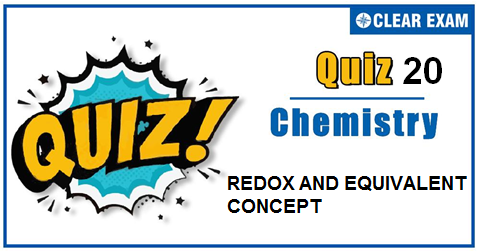## REDOX AND EQUIVALENT CONCEPT QUIZ-20

The one subject in NEET which is candidates who can easily attain good marks is Chemistry. That's the reason, often, one doesn't pay notice and choose to compromise it. But if one wants to rank above others, the tip is to be thorough with NEET chemistry concepts. The understanding of reactions and definite basic understanding is what requires major attention in Chemistry but once done it only gets simpler from there. The main focus on the to-do list should be on getting a hang of the NCERT syllabus of NEET chemistry. .

Q1. In which of the following compounds, the oxidation number of iodine is fractional?
•  IF3
•  IF5
•  I3-
•  IF7
Solution
Oxidation number of iodine in given species is as follows O.N. of iodine in IF3=+3 O.N. of iodine in I3-=-1/3 O.N. of iodine in IF5=+5 O.N. of iodine in IF7=+7

Q2.How many electrons are involved in oxidation of KMnO4 in basic medium?
•  1
•  2
•  5
•  3
Solution
Q3.  Which reaction indicates the oxidising behavior of H2 SO4?
•   2PCl5+H2 SO4 ⟶2POCl3+2HCl+SO2 Cl2
•  2NaOH+H2 SO4 ⟶Na2 SO4+2H2 O
•  NaCl+H2 SO4 ⟶NaHSO4+HCl
•  2HI+H2 SO4 ⟶I2+SO2+2H2 O
Solution
2e+S(6+) ⟶S(4+) S of H2 SO4 is reduced.

Q4. The best oxidising agent of the oxygen family is:
•  Tellurium
•  Selenium
•  Sulphur
•  Oxygen
Solution
Oxygen has highest electron affinity in its family.

Q5.In sodium hydride, oxidation state of sodium is :
•  Zero
•  +1
•  -1
•  +2
Solution
In ionic hydrides, H has -1 ox.no.

Q6. Oxidation number of ‘N’ in N3 H(hydrazoic acid) is
•  -1/3
•  +3
• 0
•  -3
Solution
N3 H (hydrazoic acid) +3(x)+1=0 3x+1=0 x=-1/3

Q7. The eq. wt. of Fe3 O4 in , Fe3 O4 + KMnO4 ⟶ Fe2 O3 + MnO2 is:
•  M/6
•  M
•  2M
•  M/3
Solution
2e+2Fe3(8/3)+)⟶ 3Fe2(3+) ∴E(Fe3 O4 =M/(No.of electrons lost or gained by one molecule) = M/1

Q8.The oxidation number of iodine in IF5 is :
•  +5
•  -5
•  -1
•  +1
Solution
a+5 × (-1)=0 ∴a= +5

Q9.The eq. wt. of FeC2 O4 in , FeC2 O4 ⟶Fe(3+)+2CO2 is∶
•  its mol. wt.
•  mol. wt./3
•  mol. wt./4
•  None of these
Solution
Fe(2+) ⟶Fe(3+)+e ( (C(3+) )2⟶2C(4+)+2e)/(Fe(2+)+C2 O4(2-)⟶CO2+Fe(3+)+3e)_ ∴E=M/3

Q10. Which compound has oxidation number of carbon equal to zero?
•  C6 H6
•  CH3
•  C2 H4
• C6 H12 O6
Solution
6 × a+12 × 1+6 × (-2)= 0 ∴a=0## Want to know more

Please fill in the details below:

## Latest IITJEE Articles$type=three$c=3$author=hide$comment=hide$rm=hide$date=hide$snippet=hide ## Latest NEET Articles$type=three$c=3$author=hide$comment=hide$rm=hide$date=hide$snippet=hide

Name

ltr
item
BEST NEET COACHING CENTER | BEST IIT JEE COACHING INSTITUTE | BEST NEET & IIT JEE COACHING: REDOX AND EQUIVALENT CONCEPT QUIZ-20
REDOX AND EQUIVALENT CONCEPT QUIZ-20